StatLect

# Linear regression - Model selection criteria

How do we choose among different linear regression models? How do we decide whether to use a more parsimonious model or one that includes several regressors?

This kind of choice is often performed by using so-called information criteria, which we briefly discuss in this lecture.## Information criteria

Information criteria are used to attribute scores to different regression models.

A score is:

• decreasing in the fit of the model (the better the model fits the data, the lower the score);

• increasing in the complexity of the model (the more regressors and parameters, the higher the score).

The best model is the one with the lowest score.

## Rationale

Generating a trade-off between fit and complexity discourages overfitting, that is, the tendency of complex models to fit the sample data very well and make poor predictions out of sample.

## Notation

In what follows,is the sample size,is the number of regressors andis the sum of squared residuals:whereis the dependent variable,is thevector of regressors, andis the OLS estimate of thevector of regression coefficients.

## The sum of squared residuals

The productis the prediction ofand the differenceis the prediction error or residual.

By squaring the residuals and summing them up, we obtain the sum of squared residuals.

The largeris, the worse the fit of the model.

## Popular information criteria

We now list some popular information criteria:

• Akaike Information Criterion (AIC):• Corrected Akaike Information Criterion (AICc):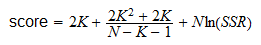• Hannan-Quinn Information Criterion (HQIC):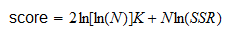• Bayesian Information Criterion (BIC):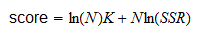## How the criteria work

All of the criteria are increasing in: the larger, the higher the score.

They are also increasing in: the larger the number of parameters (and the more complex the model), the higher the score.

However, while an increase inhas always the same effect on the score, an increase inhas different effects, depending on the criterion.

The criteria are ordered based on the strength of the penalty for model complexity: the AIC imposes the mildest penalty, while the BIC has the strongest one.

## Example

Given 20 observations, we estimate a regression model with 2 regressors and we obtain a sum of squared residuals equal to 10.

Then, we find a new regressor. We add it to our regression and the sum of squared residuals decreases to 9.5.

Which of the two models is better according to the Akaike Information Criterion?

The score of the first model (2 regressors) is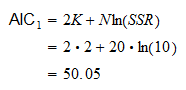The score of the second model (3 regressors) is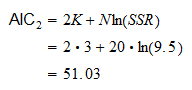The best model is the one that has the lowest score.

Therefore, the best model according to the Akaike criterion is the model with two regressors.

## How the criteria are derived

The information criteria above are used not only for linear regression, but for any statistical model estimated by maximum likelihood (ML).

The general formulae involve the log-likelihood of the model, evaluated at the ML parameter estimate.

Denote the log-likelihood by.

The general formulae (explained here) are: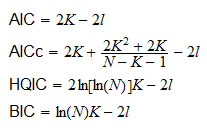The formulae for linear regression (reported previously) are obtained by making the substitutionHere is a proof that the latter is the log-likelihood of a linear regression model.

Proof

In the normal linear regression model (a model with normally distributed errors), the log-likelihood function is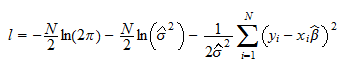whereis the OLS estimate of the vector of regression coefficients (which coincides with the ML estimate) and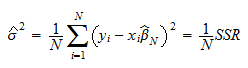is the ML estimate of the variance of the error terms. By substituting the formula forin the expression for the log-likelihood, we get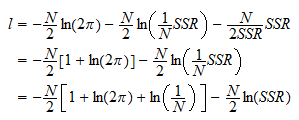We can add or subtract a constant to the scores provided by an information criterion without changing the ranking of the models. Therefore, we can drop the constant and write## Which criterion to use

Is there a preferred criterion? For example, is Hannan-Quinn better than Akaike?

There are many papers that compare the various criteria. What they find is that their performance in selecting the best model is very much dependent on the specific application.

Therefore, analysts and researchers tend to use many criteria simultaneously and report all of them.

If all the criteria select the same model, then there is little room for doubt.

On the contrary, if different criteria select different models, the interpretation is that there is no clear winner. Then, the choice can be made on other grounds, for example:

• we choose the most parsimonious model because we have a preference for simplicity;

• we select the model that includes a certain regressor because we have prior information about the importance of that regressor;

• we pick the model that has other desirable properties (e.g., well-behaved residuals).

## Alternative

An alternative to using information criteria is to check the out-of-sample predictive ability of different models.

This is usually done with cross-validation techniques (e.g., holdout, k-fold and leave-one-out).